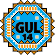General Utility Library for C++14  2.9
gul14::StandardDeviationMean< DataT, typename > Class Template Reference

## Detailed Description

### template<typename DataT, typename = std::enable_if_t<std::is_floating_point<DataT>::value>> class gul14::StandardDeviationMean< DataT, typename >

A struct holding a standard deviation and a mean value.

DataT must be a floating point type.

Default constructed the contained values are Not-a-Number.

The object can be cast to DataT in which case it is results in the sigma value. DataT is usually statistics_result_type.

The data members are public to allow structured bindings.

Template Parameters
 DataT Type of the contained values
standard_deviation()

`#include <statistics.h>`

## Public Member Functions

operator DataT () const noexcept
Cast to DataT results in standard deviation value. More...

auto sigma () const noexcept -> DataT
Get the standard deviation value.

auto mean () const noexcept -> DataT
Get the arithmetic mean value.

## Public Attributes

DataT sigma_ { NAN }
The standard deviation (sigma) value.

DataT mean_ { NAN }
The mean value.

The documentation for this class was generated from the following file: